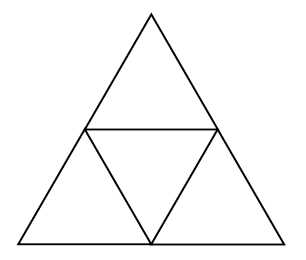### Home > CCA > Chapter 5 > Lesson 5.1.1 > Problem5-17

5-17.

The dartboard shown at right is in the shape of an equilateral triangle. It has a smaller equilateral triangle in the center, which was made by joining the midpoints of the three edges. If a dart hits the board at random, what is the probability that:

1. The dart hits the center triangle?

How many smaller triangles make up the bigger triangle?

The probability is $\frac{1}{4}$.

2. The dart misses the center triangle but hits the board?

How many smaller triangles can be hit if the center one is missed?